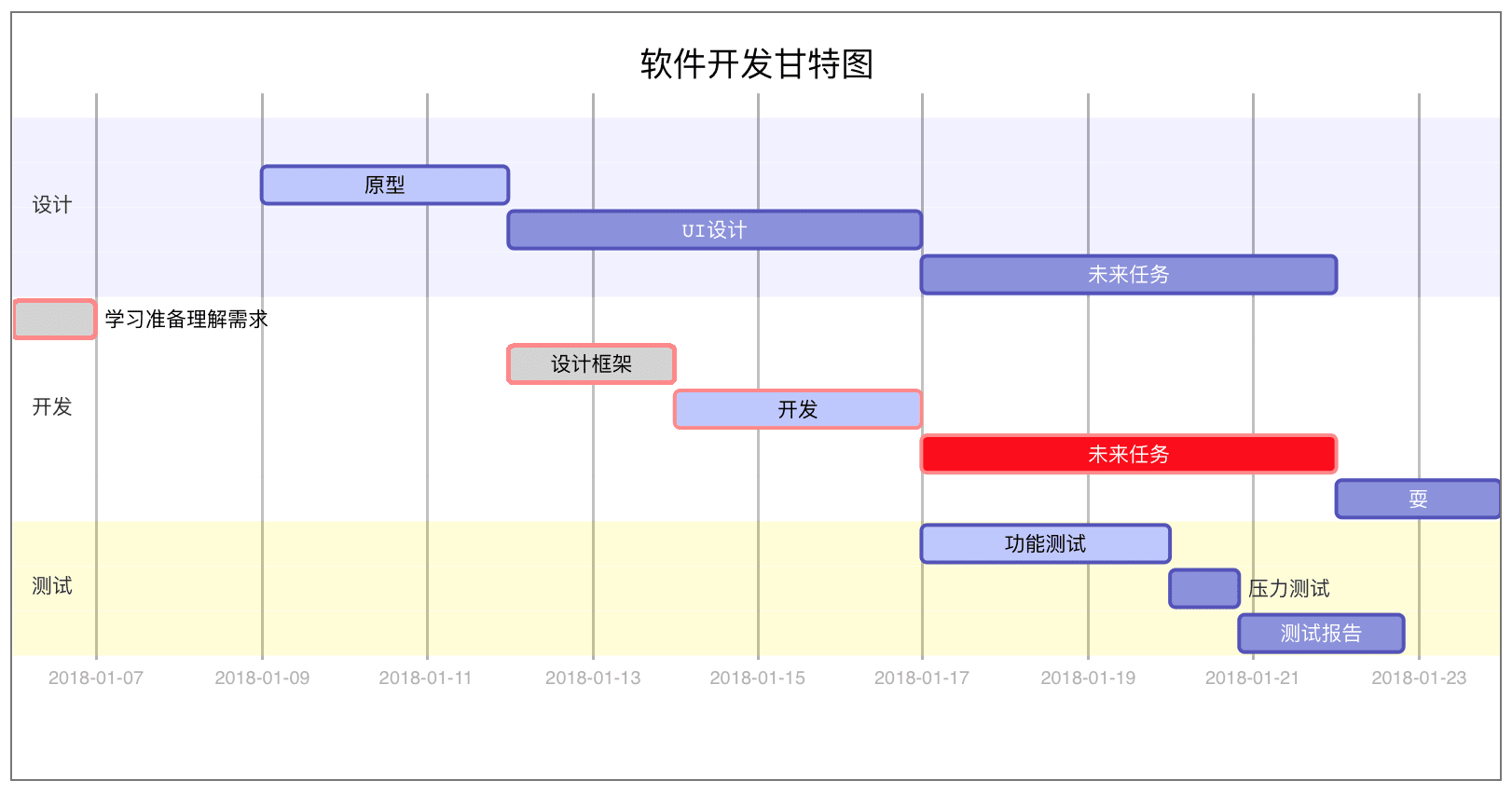# 使用markdown画流程图、时序图

## UML时序图

`hexo`中需要配合使用`hexo-filter-sequence`以及`hexo-filter-mermaid-diagrams`插件支持渲染，具体参考：Hexo常用插件

``````npm install --save hexo-filter-mermaid-diagrams
npm install --save hexo-filter-sequence
npm install --save hexo-filter-flowchart``````

### 简单示例

``````\`\`\`sequence
A->B: Hello B(请求)?
Note right of B: 对B的一些描述信息
Note left of A: 对A的一些描述
B-->A: I'm find(响应)!两杠是虚线
A->B: I'm really find.
B-B: I'm touch myself.
\`\`\```````

``````A->B: Hello B(请求)?
Note right of B: 对B的一些描述信息
Note left of A: 对A的一些描述
B-->A: I'm find(响应)!两杠是虚线
A->B: I'm really find.
B-B: I'm touch myself.``````

### 复杂示例

``````\`\`\`sequence
Title: 使用：复杂使用
A->B: Hello B?（请求）
Note right of B: 对B的描述
Note left of A: 对A的描述(提示)
B-->A: I'm Find!(响应)
B->C: How are you?
C-->>A: B touch me
A->B: are you sure？
Note over C,B: We are friend
participant C
Note right of C: 没人陪我玩
\`\`\```````

``````Title: 使用：复杂使用
A->B: Hello B?（请求）
Note right of B: 对B的描述
Note left of A: 对A的描述(提示)
B-->A: I'm Find!(响应)
B->C: How are you?
C-->>A: B touch me
A->B: are you sure？
Note over C,B: We are friend
participant C
Note right of C: 没人陪我玩``````

### 标准示例（使用mermaid标签）

``````\`\`\`mermaid
%% 时序图例子,-> 直线，-->虚线，->>实线箭头

sequenceDiagram

participant 张三

participant 李四

loop 健康检查

end

Note right of 王五: 合理 食物 <br/>看医生...

\`\`\```````

``````%% 时序图例子,-> 直线，-->虚线，->>实线箭头

sequenceDiagram

participant 张三

participant 李四

loop 健康检查

end

Note right of 王五: 合理 食物 看医生...

## 流程图

### 横向流程图

``````\`\`\`mermaid
graph LR

A[方形] -->B(圆角)

B --> C{条件a}

C -->|a=1| D[结果1]

C -->|a=2| E[结果2]

F[横向流程图]
\`\`\```````

``````graph LR

A[方形] -->B(圆角)

B --> C{条件a}

C -->|a=1| D[结果1]

C -->|a=2| E[结果2]

F[横向流程图]``````

### 纵向流程图

``````\`\`\`mermaid
graph TD

A[方形] -->B(圆角)

B --> C{条件a}

C -->|a=1| D[结果1]

C -->|a=2| E[结果2]

F[竖向流程图]
\`\`\```````

``````graph TD

A[方形] -->B(圆角)

B --> C{条件a}

C -->|a=1| D[结果1]

C -->|a=2| E[结果2]

F[竖向流程图]``````

### 标准流程图(flow)

``````\`\`\`flow
st=>start: 开始框
op=>operation: 处理框
cond=>condition: 判断框(是或否?)
sub1=>subroutine: 子流程
io=>inputoutput: 输入输出框
e=>end: 结束框
st->op->cond
cond(yes)->io->e
cond(no)->sub1(right)->op
\`\`\```````

``````st=>start: 开始框
op=>operation: 处理框
cond=>condition: 判断框(是或否?)
sub1=>subroutine: 子流程
io=>inputoutput: 输入输出框
e=>end: 结束框
st->op->cond
cond(yes)->io->e
cond(no)->sub1(right)->op``````

## 甘特图

``````\`\`\`mermaid
%% 语法示例

gantt

dateFormat  YYYY-MM-DD

title 软件开发甘特图

section 设计

UI设计                     :         des3, after des2, 5d

section 开发

section 测试

\`\`\```````